6. Value Solids and Multi-Valued Logics

A slight variation in the representation of the value spaces outlined in section 5. offers an intriguing comparison with a way of envisaging connectives in multi-valued logics, including infinte-valued or fuzzy logics.

Rather than graphing values in our value space in terms of color, the use of binary decimals makes it easy to graph them in terms of height in a third dimension. A value of .0000 will graph as 0, a value of .1000 as the decimal equivalent .5, .1100 as .75, and so forth.Fig. 27
A fairly rough graph of this sort for NAND, seen from a particular angle, appears in figure 27. This corresponds directly to Figure 20, though here the origin is in the right rear corner. Smoother forms of the value solid for NAND, from two angles, appear in figure 28. Because the rough solids are often more revealing of basic structure, however, we will continue with these throughout.AND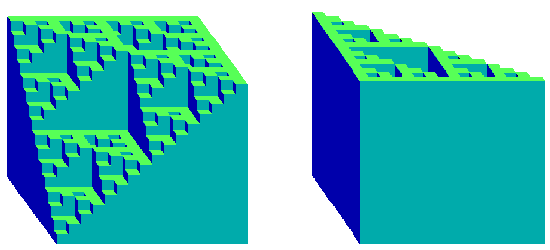ORIMPLICATION

Fig. 29

Value solids for conjunction, disjunction, and material implication appear in Fig. 29. In each case the origin is shown in the left figure at the front left, and in the right figure at the rear right.

These value solids make obvious the relationships between NAND and OR, the dual character of conjunction and disjunction, and the rotation properties of negation. Of perhaps deeper significance, however, these value solids for simple classical systems also show a striking resemblance to a very different type of solid that can be drawn for connectives within multi- valued or infinite-valued logics.

In this second type of solid, values on the axes represent not truth-table columns but degrees of truth. Within the value solid, height at a certain point represents the degree of truth of a complex of two sentences with the axis values of that point. In one standard treatment of infinite-valued connectives, for example, the value of a conjunction of sentences p and q is the minimum value of the two, represented as:

/p&q/ = min(p,q).
The value solid of this type for conjunction will thus at each point have a height corresponding to the minimum of its axis values.

There are, however, rival sets of connectives that have been proposed for multi-valued and infinite-valued or fuzzy logics. One such set, perhaps most common within multi-valued and fuzzy logics, is shown in the left column of Fig. 30. Another set, grounded more directly in the original multi-valued logic ofukasiewicz, is shown in the right column.

AND
`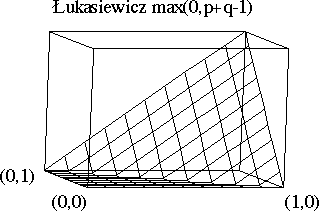`

OR
`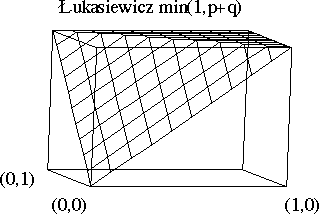`

Implication
``

Fig. 30

It should be emphasized that the value solids appropriate to connectives in infinite-valued logic are radically different from the value-solids for systems outlined above. In system value solids, for example, .1000 might represent a truth-table in which the first line has a 'T' and the others do not. In that regard it is perfectly symmetrical to .0001, which simply has a 'T' on a different line. Using similar binary decimals for the values of sentences in an infinite-valued logic, on the other hand, a statement with the value .1000 would be half true. One with a value of .0001 would be almost completely false.

Given that radical difference, the value solids outlined here for classical systems and those sketched above for infinite-valued logics seem much more alike than they have any right to be. Intriguingly, the system-solid for each connective seems to be embody a compromise between the corresponding infinite-valued connective solids. The system-solid for 'or', for example, amounts neither to 'max' nor to theukasiewicz 'or'. It rather appears to be a compromise, in which some values correspond to one treatment of the infinite-valued connective and one to another.

Indeed this is precisely what is happening. How it occurs--and why there is such a resemblance between these two radically different kinds of value solid--becomes clear if we return to two dimensions and consider a simple form of our basic value grid.

In a system grid for 'or', in which we are calculating the truth-table values for an 'or' between truth-table values on the axes, the value assigned to any intersection point is what might be called a 'bitwise or' of the values on the corresponding axes. A '1' occurs in any row in the value of that intersection point just in case a '1' occurs in that row in one or the other of the corresponding axis values. In bitwise 'or' the 1's cannot of course add together and carry to another row:

 p q (p or q) 1 1 0 0 1 0 1 0 1 1 1 0
The values assigned in a system grid for 'or', then, correspond to a bitwise 'or'. The values assigned to intersection points in an infinite-valued grid will be more complicated, amounting to either the maximum of the axis values p and q or, in the case of theukasiewicz 'or', to min (1, p+q). Nonetheless these three values for intersection points will occasionally overlap.

In the simple case of three-digit binary decimals, in fact, where we take 111 as the closest approximation to 1 in theukasiewicz formula, every bitwise 'or' is equal to either max, theukasiewicz 'or', or both. This is reflected in the 8 by 8 grids shown in Figure 31. On the first figure those intersection points are mapped in which a bitwise 'or' corresponds to 'max'. On the second are mapped those intersection points in the grid in which bitwise 'or' corresponds to theukasiewicz 'or'. Here it is clear (a) that the middle areas, exclusive of the edges, are the negatives of each other, (b) that together these two graphs will therefore cover the entire area of the grid, and (c) that each middle area represents a simple Sierpinski gasket, rotated 90 degrees from the other graph. A value solid for bitwise 'or' geared to just three-digit binaries, then, would at each intersection point correspond precisely to one or the other of the two infinite-valued connectives outlined above: the 8-valued system solid constitutes a perfect Sierpinski compromise between the two infinite-valued solids.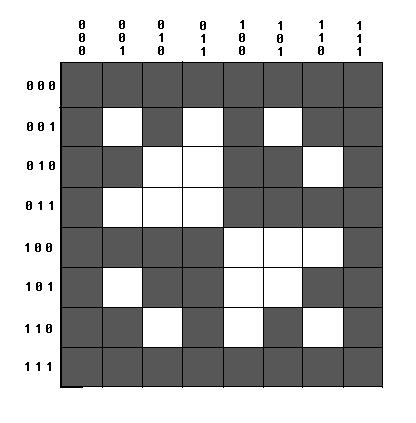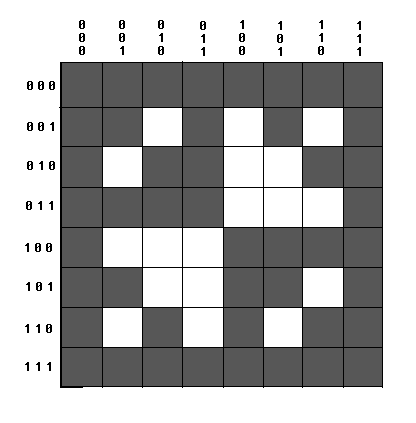Fig. 31

The result does not generalize in this pure form to system- and infinite-valued solids of more than eight units on a side, however. In more complex cases the Sierpinski patterns persist, but their overlap fails to cover the entire area. For a grid of 256 values on each side, Figure 32 shows in black those intersection points in which bitwise 'or' will equal one or the other of our two infinite-valued connectives. The holes left are the holes formed by one Sierpinski gasket overlying another rotated at 90 degrees. Even in more complex systems a type of compromise remains, however. For in all cases the bitwise 'or' for an intersection point will equal either one of the two infinite-valued 'or's above or will have a value between them, less than theukasiewicz but greater than simply 'max'. Similar compromises hold in the case of the other connectives. Thus in an intriguing way value-solids for simple systems do map a compromise between the quite different value-solids appropriate to rival connectives within infinite-valued or fuzzy logic.Fig. 32